• 不变系统 因果系统 稳定系统 系统跳过了电路的具体结构，只考虑输入(激励e(t)e(t)e(t))和输出（响应r(t)r(t)r(t)）的关系。 其中系统函数H[n]H[n ]H[n]是表征系统固有特性的函数，因此与外加激励毫无关系，只...

系统的分类

根据不同的角度，可以把系统分成不同的种类：
连续系统和离散系统
即时系统与动态系统
集总参数系统和分布参数系统
可逆系统与不可逆系统
线性系统
时不变系统
因果系统
稳定系统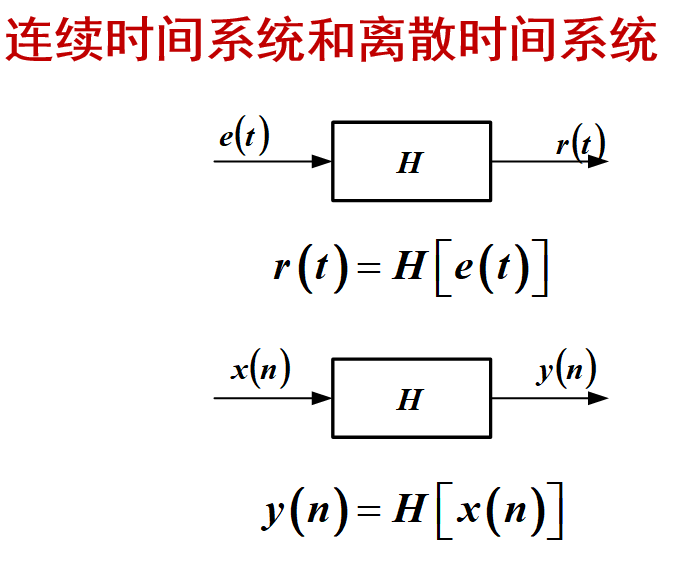系统跳过了电路的具体结构，只考虑输入(激励$e(t)$)和输出（响应$r(t)$）的关系。
其中系统函数$H[n ]$是表征系统固有特性的函数，因此与外加激励毫无关系，只与系统的固有结构有关。
外加激励默认在零时刻加进去，系统零时刻之前的原始储能状态为初始状态。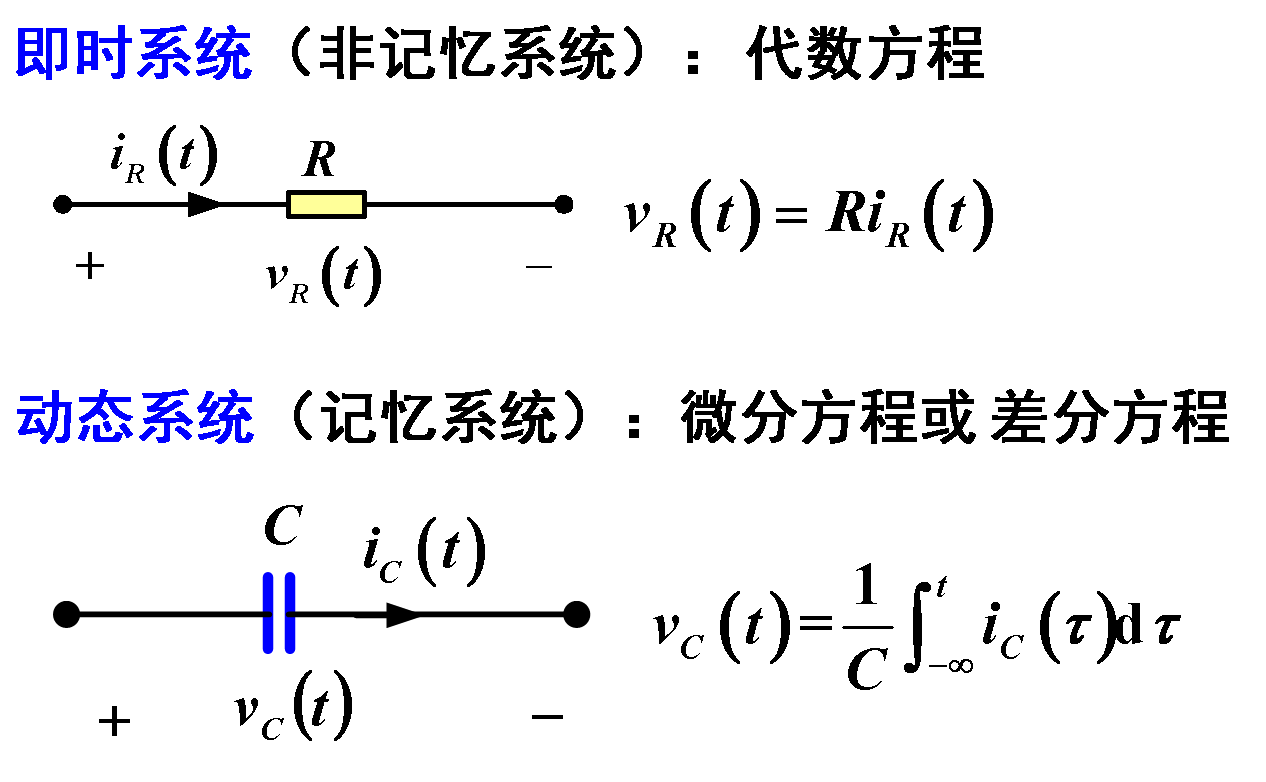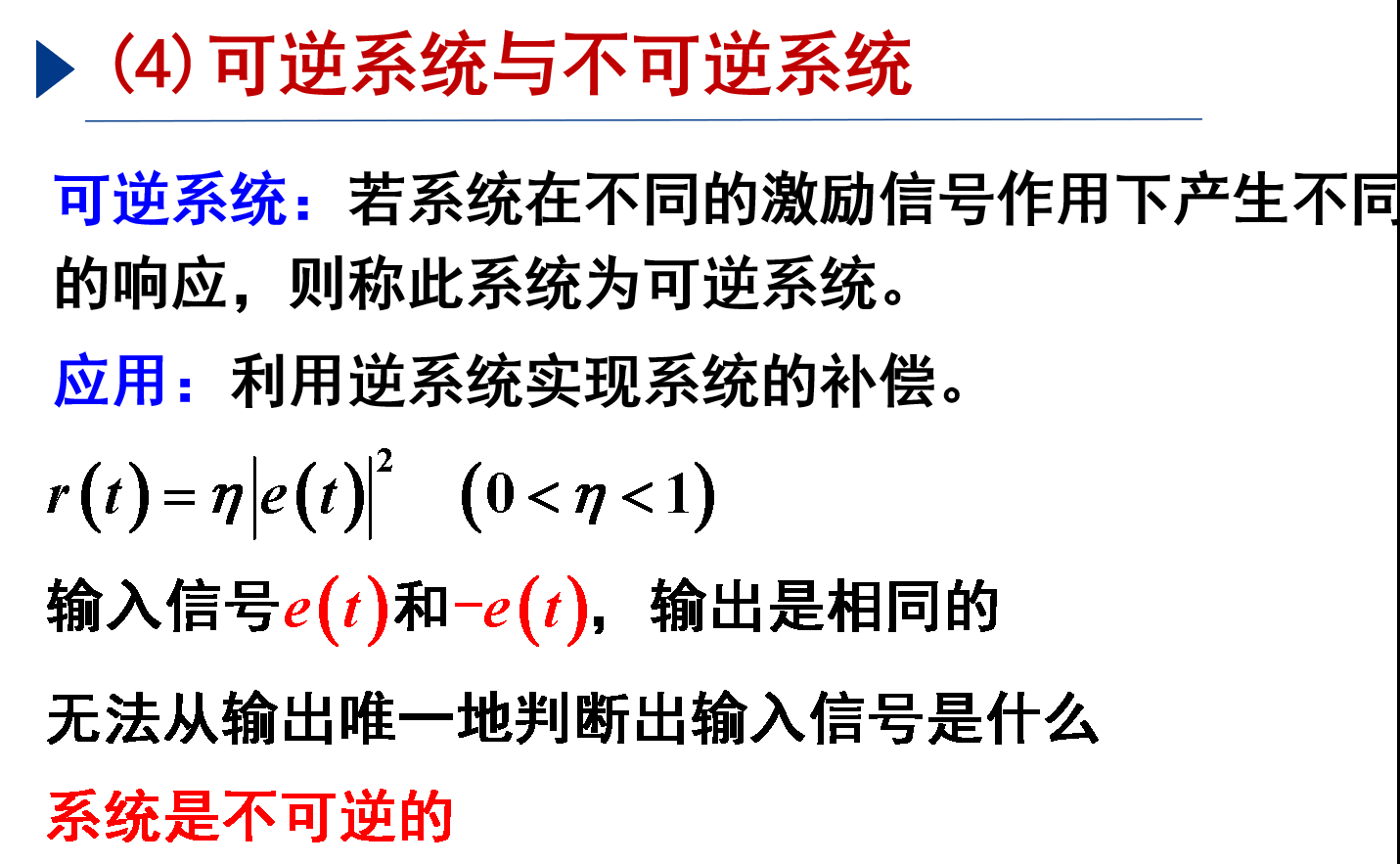倒推推出来是一对一的是可逆，确定不了是一对多的是不可逆

线性系统

线性指的是均匀性（齐次）和叠加性（可加），两者同时满足才可称为线性，否则就是非线性。
判断一个系统是否为线性：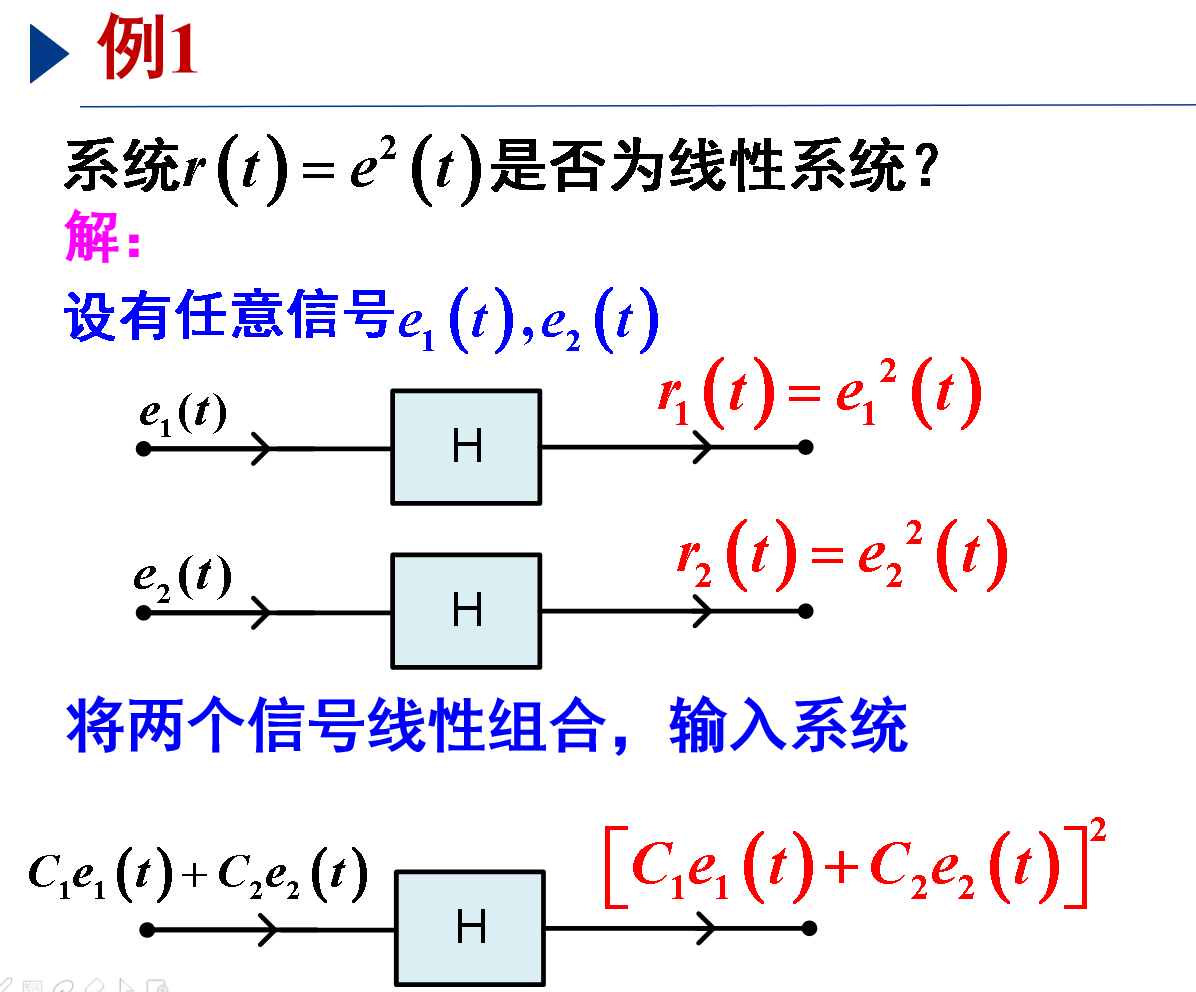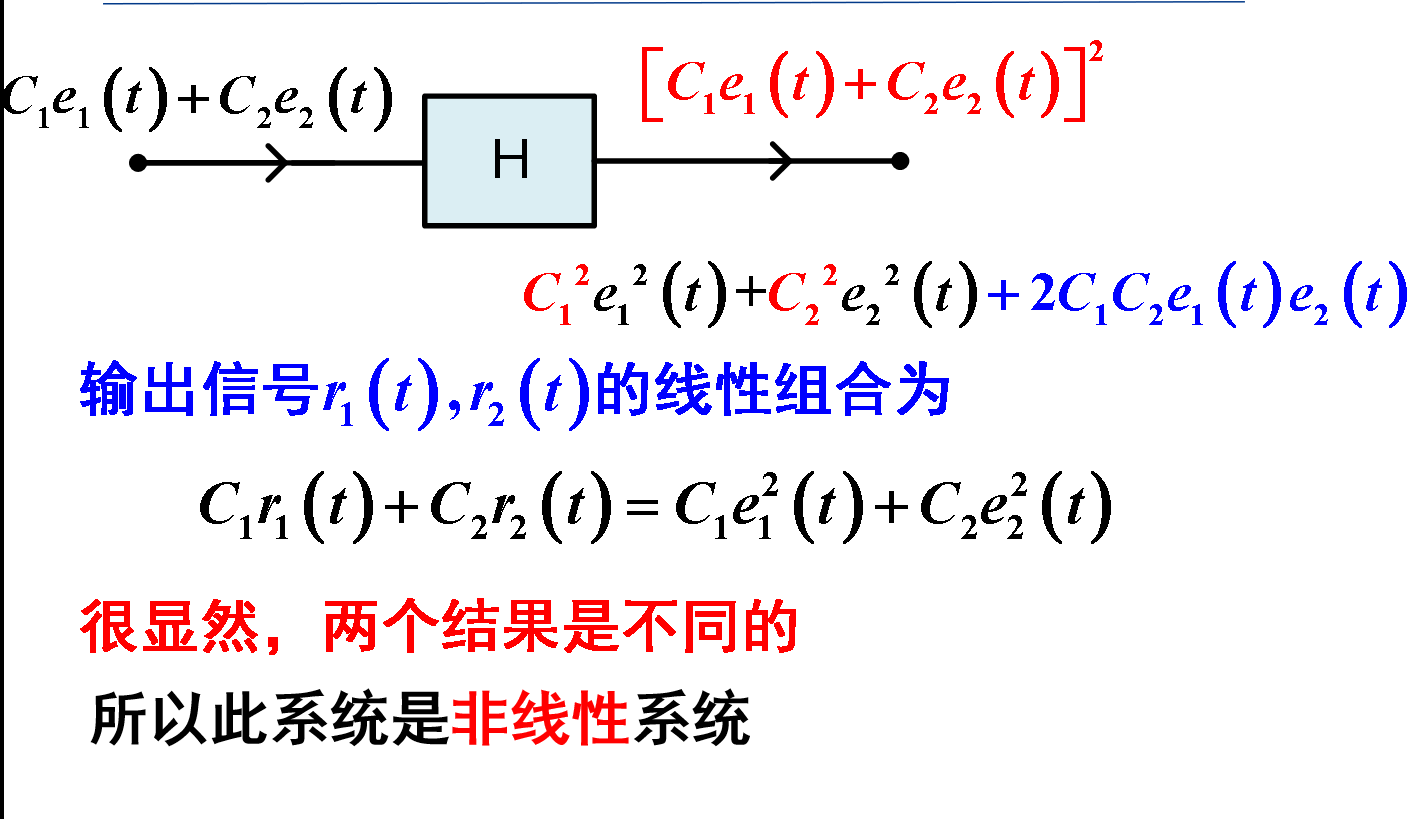把原来的激励信号和把原来的响应当作新的激励信号分别经过线性组合之后看是否等价，是就是线性，不是就是不是。

时不变系统（非时变）

以微分电路为例：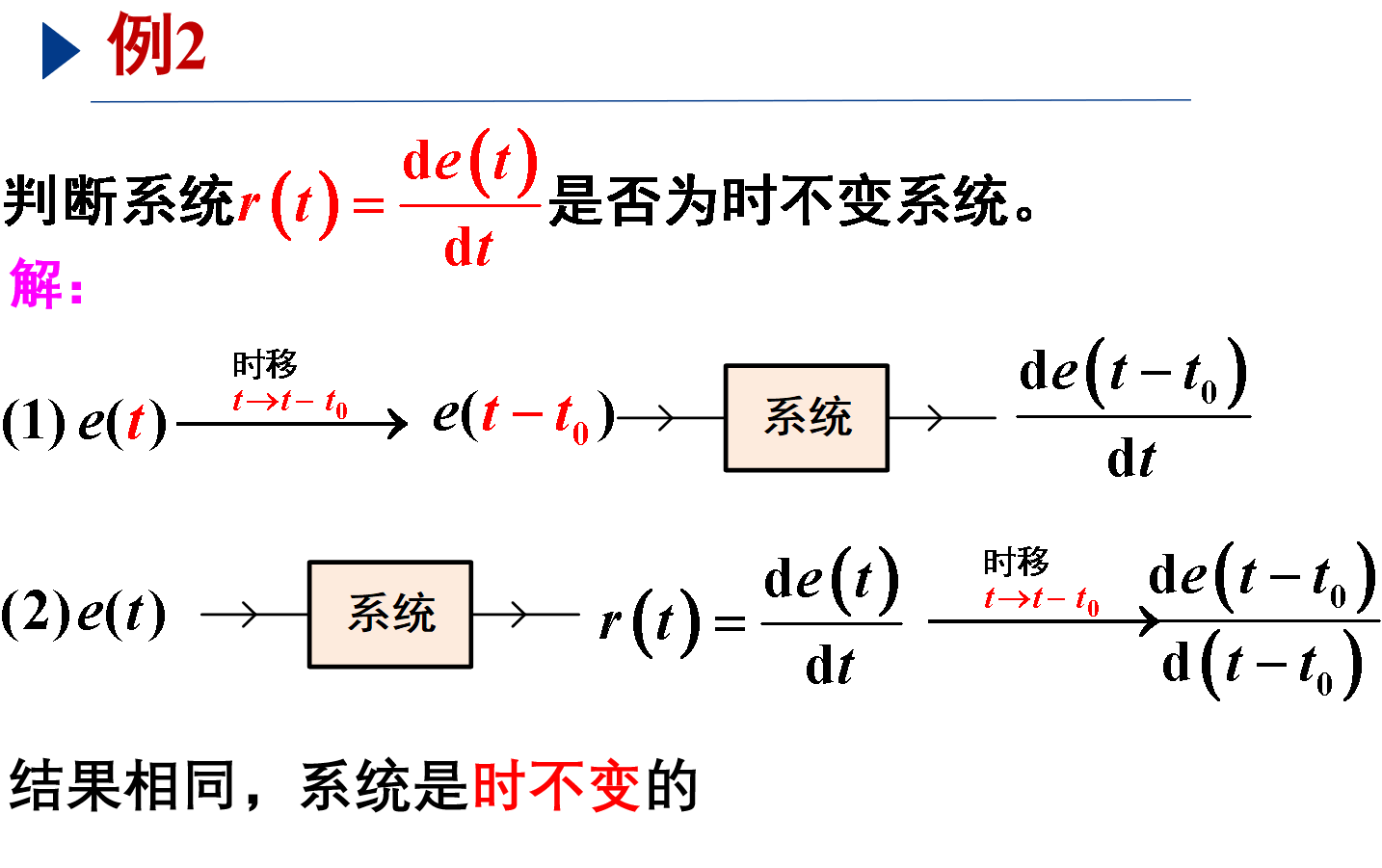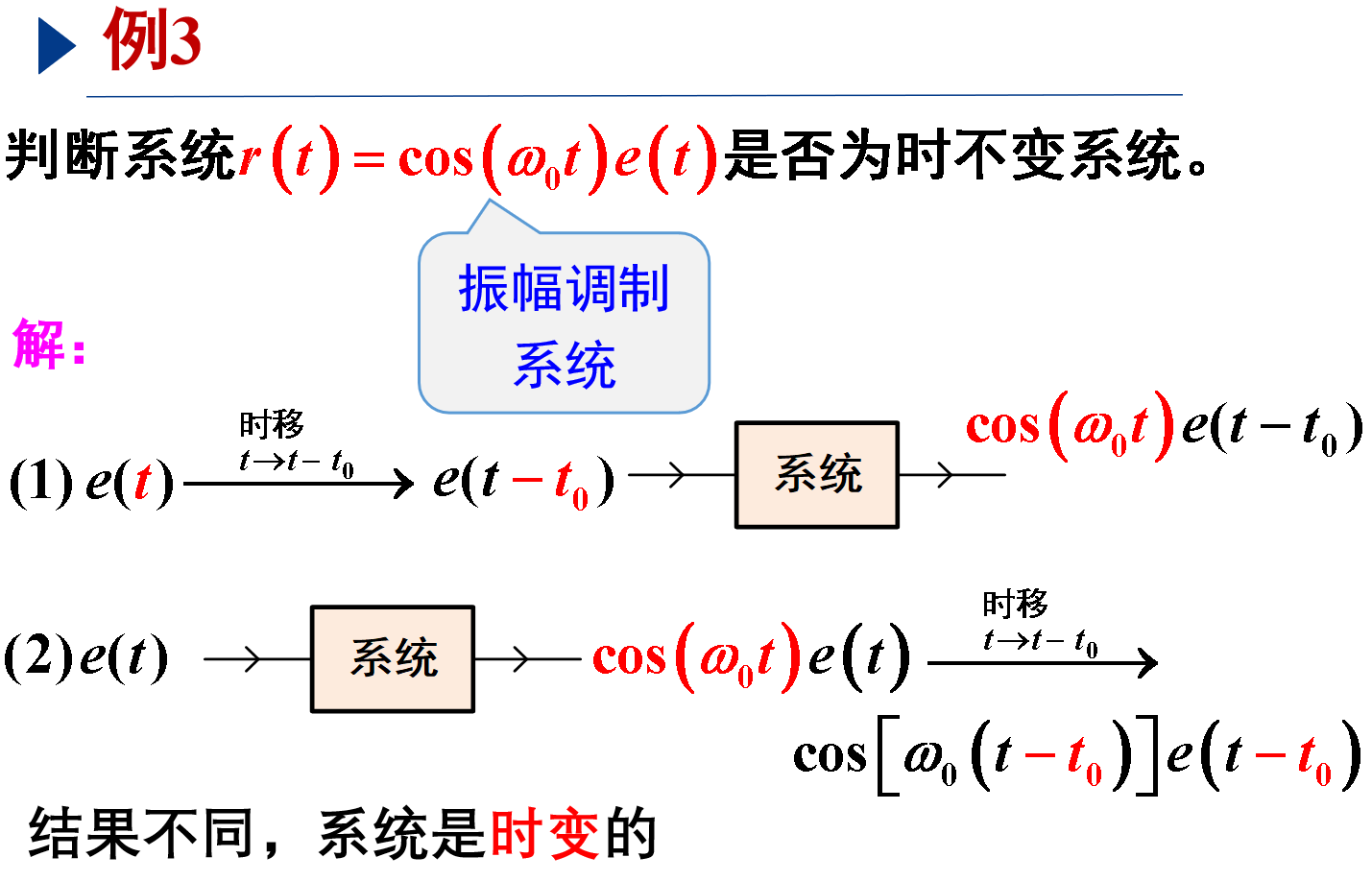分两步：
(1) 将原来的激励信号先时移，再经过系统的响应得到的结果
(2) 将原来的激励信号先经过系统的响应，再时移得到的结果
如果（1）和（2）的结果相同就是时不变，否则为时变。

Linear and Time-invariant System 线性时不变系统（LTI）

LTI性质：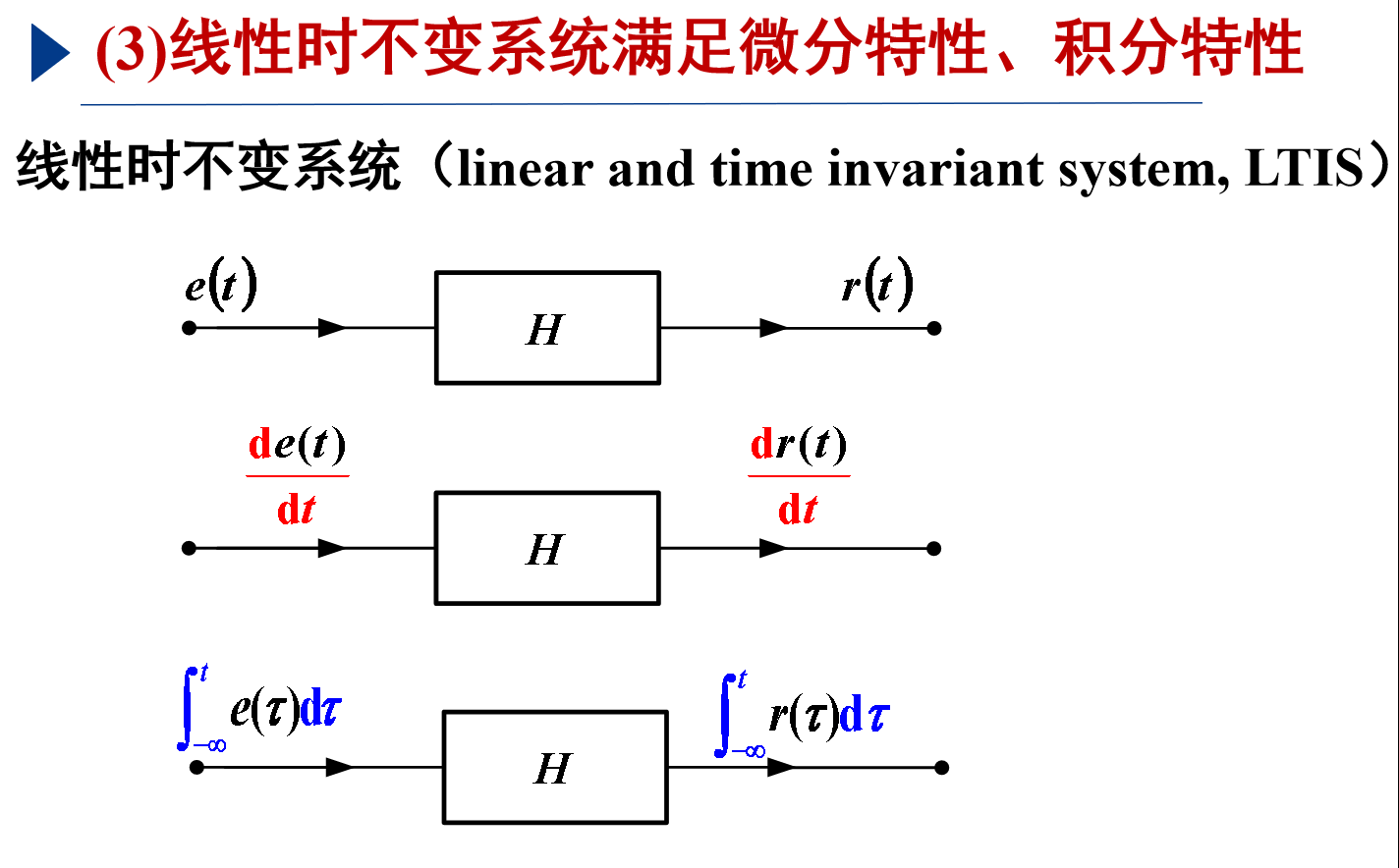微积分性质可以推广至高阶

因果系统

系统的因果关系：从系统的因果性来看，输入（激励）是输出（响应）的原因，输出是输入的结果。系统的因果信号：借助“因果”这一关系，我们称在t=0之后对系统产生影响的信号为因果信号，换句话说，t<0时，信号取值为0的信号为因果信号。

目前物理可实现的系统都是因果系统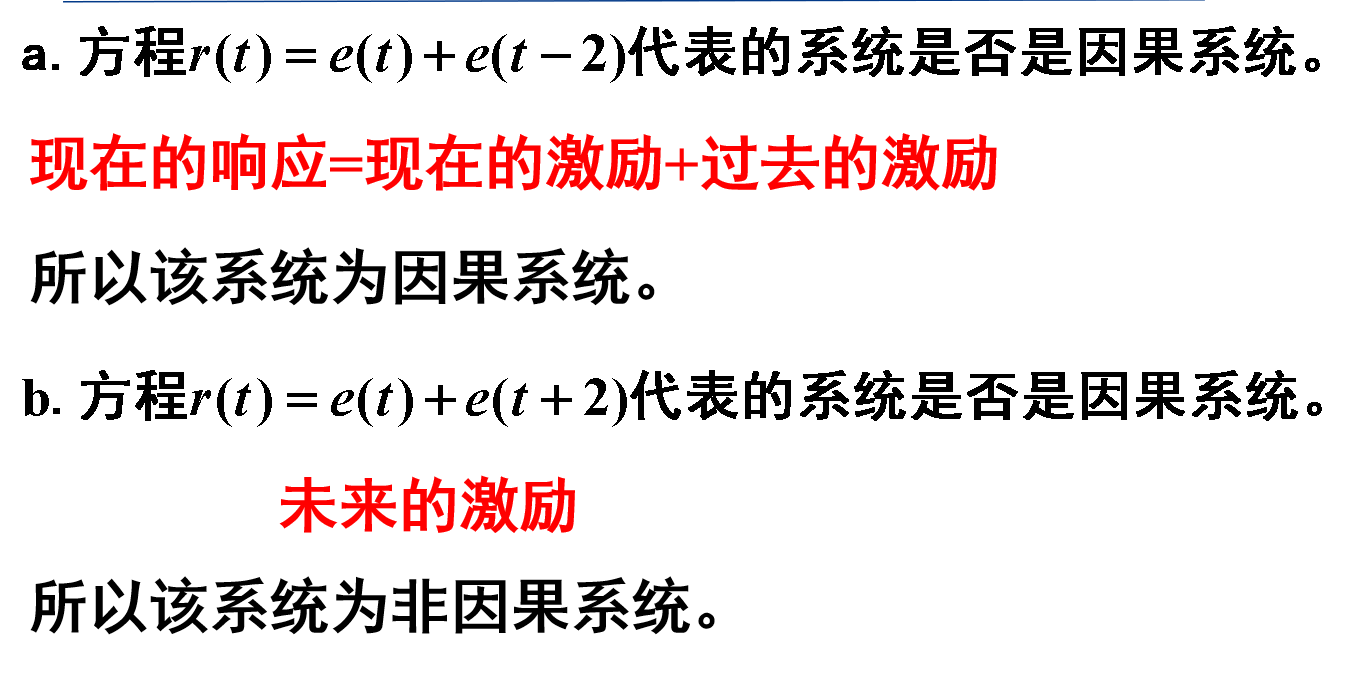如果$t$前的加权系数不为1就不能这么判断了
例：$\bm{r(t)=e(2t)}$
因为：若$e(t)=0, 有r(t_0)=e(2t_0)=0|_{t_0=0.5},t_0<0.5t_0$，故为非因果系统。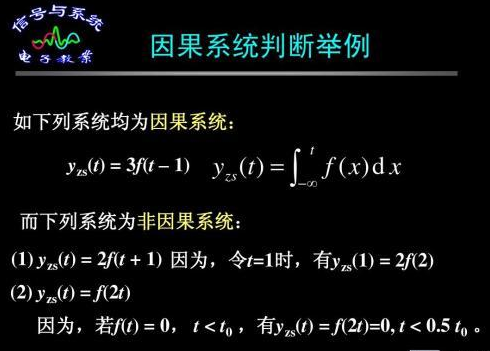需注意的是：因果信号与因果系统不是一个东西。
满足$\bm{e(t) = e(t)u(t)}$（单边信号）为因果信号。
通常在信号与系统中用到的就是这种单边拉普拉斯变换（有时也将t=0_考虑进去），也就是因果信号（含有输入信号和输出信号的信号系统）的拉氏变换。
详情可参考拉普拉斯变换【直观解释】—复变函数与积分变换学习笔记

因果信号与因果系统

总之，线性时不变系统是最基础的最简单的系统，相应的非线性时变系统最为复杂，


展开全文• 也就是说,若y[n]y[n]y[n]是一个离散时间时不变系统在输入为x[n]x[n]x[n]时的输入,那么当输入为x[n−n0]x[n−n0]x[n-n_0]时,输入就是y[n−n0]y[n−n0]y[n-n_0]. 线性:令y1(t)y1(t)y_1(t),y2(t)y2(t)y_2(t)分别...
定义

时不变性:如果在输入信号上有一个时移,而在输出信号中产生同样的时移,那么这个系统就是时不变的.也就是说,若y[n]$y\left[n\right]$$y[n]$是一个离散时间时不变系统在输入为x[n]$x\left[n\right]$$x[n]$时的输入,那么当输入为x[n−n0]$x\left[n-{n}_{0}\right]$$x[n-n_0]$时,输入就是y[n−n0]$y\left[n-{n}_{0}\right]$$y[n-n_0]$.
线性:令y1(t)${y}_{1}\left(t\right)$$y_1(t)$,y2(t)${y}_{2}\left(t\right)$$y_2(t)$分别为连续时间系统对x1(t)${x}_{1}\left(t\right)$$x_1(t)$,x2(t)${x}_{2}\left(t\right)$$x_2(t)$的响应,则对一个线性系统,有:
y1(t)+y2(t)${y}_{1}\left(t\right)+{y}_{2}\left(t\right)$$y_1(t)+y_2(t)$是对x1(t)+x2(t)${x}_{1}\left(t\right)+{x}_{2}\left(t\right)$$x_1(t)+x_2(t)$的响应
ay1(t)$a{y}_{1}\left(t\right)$$ay_1(t)$是对ax1(t)$a{x}_{1}\left(t\right)$$ax_1(t)$的响应,其中a$a$$a$为任意复常数
无记忆系统:一个系统的输出仅仅取决于该时刻的输入,这个系统就称为无记忆系统
因果性:如果一个系统在任何时刻的输出只取决于现在的输入和过去的输入,则该系统就称为因果系统
奇异函数:函数本身有不连续点(跳跃点)或其导数和积分有不连续点的一类函数.

卷积和

公式

离散系统
y[n]=∑k=−∞+∞x[k]h[n−k]=x[k]∗h[k](1)$\begin{array}{}\text{(1)}& y\left[n\right]=\sum _{k=-\mathrm{\infty }}^{+\mathrm{\infty }}x\left[k\right]h\left[n-k\right]=x\left[k\right]\ast h\left[k\right]\end{array}$y[n] = \sum_{k=-\infty}^{+\infty} x[k]h[n-k]=x[k]*h[k] \tag{1}
h[k]$h\left[k\right]$$h[k]$为y[k]$y\left[k\right]$$y[k]$的单位冲激响应
连续系统
y[t]=∫+∞−∞x[τ]h[t−τ]dτ=x[t]∗h[t](2)$\begin{array}{}\text{(2)}& y\left[t\right]={\int }_{-\mathrm{\infty }}^{+\mathrm{\infty }}x\left[\tau \right]h\left[t-\tau \right]d\tau =x\left[t\right]\ast h\left[t\right]\end{array}$y[t] = \int_{-\infty}^{+\infty} x[τ]h[t-τ]dτ=x[t]*h[t] \tag{2}
h[t]$h\left[t\right]$$h[t]$为y[t]$y\left[t\right]$$y[t]$的单位冲激响应

结论

一个线性时不变系统的特性可以完全由它的冲激响应来决定
展开全文• 一、用微分和差分方程描述的因果线性时不变系统1、一类极为重要的连续时间系统是其输入输出关系用线性常系数微分方程描述的系统。这种形式的方程可以用来描述范围官广泛的系统和屋里现象。2、一类极为重要的离散时间...
注：本博客是基于奥本海姆《信号与系统》第二版编写，主要是为了自己学习的复习与加深。一、用微分和差分方程描述的因果线性时不变系统1、一类极为重要的连续时间系统是其输入输出关系用线性常系数微分方程描述的系统。这种形式的方程可以用来描述范围官广泛的系统和屋里现象。2、一类极为重要的离散时间系统是其输入输出关系用线性常系数差分方程描述的系统。这种形式的方程可以用来描述许多不同过程的程序行为。一）、线性常系数微分方程1、考虑一阶微分方程：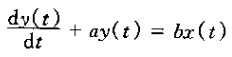其中y(t)为系统的输出，x(t)为系统的输入。这类方程所给出的是该系统的一种隐含的特性，也就是说，它们所描述的输入和输出关系并不是将系统输出作为输入函数的一种明确的表达式。2、一般来说，为了求解一个微分方程，必须给定一个或多个附加条件；一旦这些条件给定，原则上就能得到一个用输入表示输出的明确的表达式。3、一个N阶线性常系数微分方程由如下方程给出：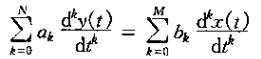阶次指的是出现在这个方程输出y(t)的最高阶导数。当N=O时，上式就变为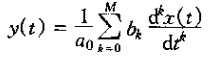此时y(t)就是输入x(t)及其导数的一个明确的函数。4、N阶线性常系数微分方程的阶由两部分组成：特解加上如下齐次微分方程的解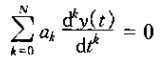此方程称为该系统的自然响应。二）、线性常系数差分方程1、离散时间N阶线性常系数差分方程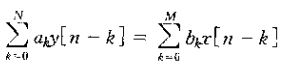上式可以用求解微分方程那样来求解。具体而言，y[n]的解可以写成特解和一个齐次方程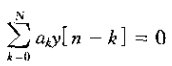2、N阶线性常系数差分方程另一种表达式为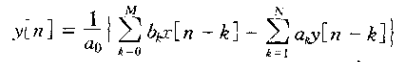上式可把n时刻的输出直接用以前输入输出值来表示；据此可立即看出需要附加条件。为了计算y[n]，就需要知道y[n-1],...,y[n-n],因此如果给出了所有n时的输入和一组附加条件，就可以连续求得个y[n]的值。两种N阶线性常系数差分方程称之为递归方程。3、在N=0的特殊情况下，上式就变成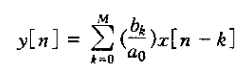此方程称为非递归方程。由上庙的方程描述了一个线性时不变系统，这个系统的单位脉冲响应为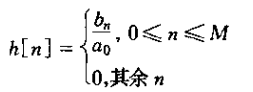此系统往往称为有限脉冲响应系统。三）、用微分和差分方程描述的一阶系统的方框图表示1、由线性常系数差分方程和微分方程描述的系统的一个重要特点是：能以很简单而且很自然的方式用若干基本运算的方框图互联来表示。这样做很有意义的：1）、给出一种形象化的表示，这有助于加深对这些系统的特性和性质的理解。2）、对于系统的仿真和实现有很大的价值。2、离散时间三种基本的网络单元：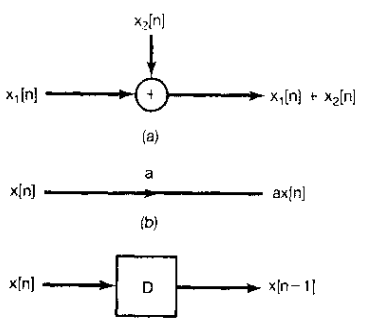3、离散时间一阶差分方程方框图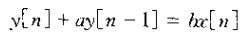方框图表示：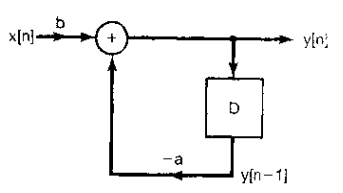4、连续时间三种基本网络单元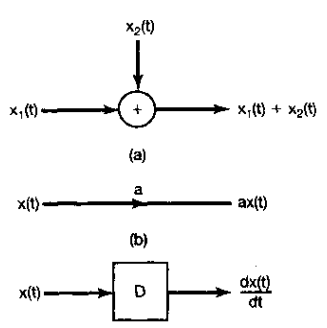5、连续时间一阶微分方程1）、不常用微分器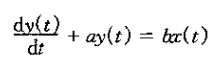2）、常用微分器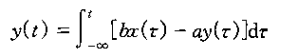3）、微分器方框图表示：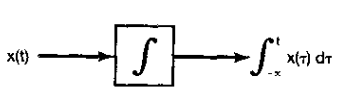4）、不常用微分器方框图表示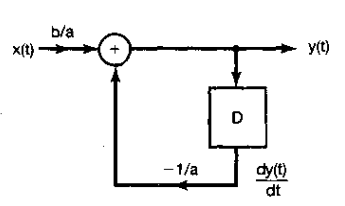5）、常用微分器方框图表示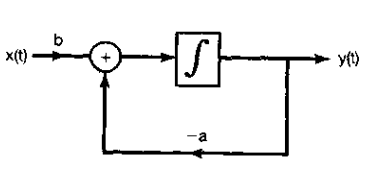二、奇异函数一）、作为理想化短脉冲的单位冲激1、如果将单位冲激定义为某信号的极限形式，那么事实上就存在着无限多个看起来很不同的洗你号，但在极限之下其表现都像一个冲激。这里所指的意思是一个线性时不变系统对所有这些信号的响应应在本质上都是一样的，只要这个脉冲“足够短”，即△"足够小"。二）、通过卷积定义单位冲激1、定义一个信号，其对任何x(t)有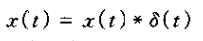由上式可以得到所需的有关单位冲激的全部性质。1）、若令x(t)=1（对所有的t），则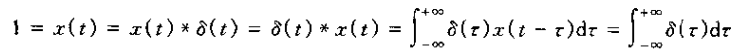所以单位冲激的面积为1.2）、取热门和信号g(t)，将其反转得到g（-t），然后再与求卷积。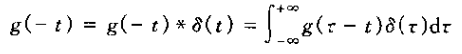对于t=0，得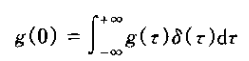上式说明：单位冲激是这样一种信号，当它与某一信号g(t)相乘并在-∞和+∞积分时，其结果就是g(0)。三）、单位冲激偶和其他奇异函数1、单位冲激是一类称为奇异函数的信号中的一种，其中每一种信号都是借助于它在卷积运算中的特性来定义的。2、考虑输出是输入的导数的线性时不变系统，即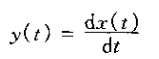这个系统的单位冲激响应是单位冲激的导数，称为单位冲激偶u1(t）。根据线性时不变系统的卷积表示，对任何信号x(t)应有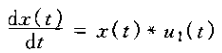可以将上式取为u1（t）的运算定义。同理也能定义二阶导数u2(t)，取输入的二阶导数的线性时不变系统的冲激响应为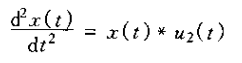一般情况下，k>0时，uk(t)就是的k此导数，因此是一个取输入k此导数系统的单位冲激响应。因为该系统可以由k个微积分级联得到，所以就有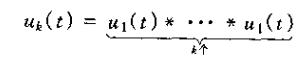3、与单位冲激一样，单位冲激函数等这些奇异函数中的每一个都可以与一些短脉冲相联系。4、除了单位冲激各不同阶导数的这些奇异函数之外，还能代表单位冲激函数连续多次积分的一些信号。
展开全文• DAY2 很多物理过程都具有线性和时不变性, 因此都能用线性时不变系统来表征. 无论在离散时间或连续时间情况下, 单位冲激函数的重要特性之一是: 一般信号都可以表示为延迟冲激的线性组合. 这个事实, 在与叠加性和时...
献给

        期末继续预习的我
还有刺猬


DAY2

    很多物理过程都具有线性和时不变性, 因此都能用线性时不变系统来表征.
无论在离散时间或连续时间情况下, 单位冲激函数的重要特性之一是: 一般信号都可以表示为延迟冲激的线性组合.
这个事实, 在与叠加性和时不变性结合起来, 就能用线性时不变的单位冲激响应来完全表征任何一个线性时不变系统的特性.
这样一种表示, 在离散时间情况下称为卷积和;
在连续时间情况下称为卷积积分;


离散时间线性时不变系统: 卷积和

    1. 用脉冲表示离散信号
如何把任何离散时间信号看成离散时间单位脉冲构成的关键是:
要把一个离散时间信号当成一串单个脉冲想象啦.

数学表示:
x[n] = Σx[k]δ[n-k] (k from -∞ to +∞) ···①
上面式子相当于把任意序列表示成一串位移的单位脉冲序列δ[n-k]的线性组合, 而这个线性组合式中的权因子就是x[k].
也体现出了离散时间单位脉冲序列的 筛选性质 :
因为δ[n-k]仅当k是n时为非零. 从而就只保留了序列x[n].

2. 离散时间线性时不变系统的单位脉冲响应及卷积和表示
式①筛选性质的重要性体现在于它把x[n]表示成一组加权的基本函数(移位单位脉冲)的叠加.
一个线性系统对x[n]的响应就是系统对这些移位脉冲中的每一个响应加权后的叠加(可以叠加是因为线性系统的特性: 可加性 & 齐次性), 而且时不变性又意味着一个时不变系统对移动单位脉冲的响应就是未被移位的单位脉冲响应的移位. 将这两点结合在一起, 即可得到具有线性和时不变性的离散时间系统的卷积和表示.
具体而言, 现在来考虑某一线性(但可能为时变的)系统对任一输入x[n]的响应. 由式①可将输入表示为一组移位单位脉冲的线性组合, 令hk[n]为该线性系统对移位单位脉冲δ[n-k]的响应, 那么根据线性系统的叠加性质, 该线性系统对输入x[n]的响应y[n]就是关于这些响应的加权线性组合.
y[n] = Σx[k]hk[n-k] (k from -∞ to +∞) ···②
由②式可知, 如果已知一个线性系统对每一个移位单位脉冲序列的响应那么系统对任何输入的响应都可求出.一个线性系统在时刻n时的响应就是在时间上每一点的输入值所产生的各个响应在该时刻n的叠加.
一般来说, 在线性系统中, 对于不同的k值, 其响应hk[n]相互之间不必有什么关系. 但是, 若该线性系统也是时不变的, 那么这些对时间移位的单位脉冲的响应也全都相互移位了:
hk[n] = h[n-k];
那么对于线性时不变系统而言
y[n] = Σx[k]h[n-k] (k from -∞ to +∞) ···③
这个结果称为卷积和(叠加和), 并用符号记为:
y[n] = x[n] * h[n];
既然一个线性时不变系统对任意输入的响应可以用系统的对单位脉冲的响应来表示, 那么线性时不变系统的单位脉冲响应就完全刻画了系统的特征. (所以, 接下来就了解了解单位脉冲~~~)
利用在每个单独输出样本上的叠加求和结果, 可以得出一种有用的方法: 用卷积和来想象y[n]的计算. 考虑一个特定的n值求y[n]的问题. 用图来展现这种计算的一种特别有用的方法是一开始就将信号x[k]和h[n-k]都看成k的函数, 将它们相乘就得到序列g[k] = x[k]h[n-k], 它可以看成在每一个时刻k, 输入x[k]对输出在时刻n做出的贡献, 这样就能得出如下结论: 将全部g[k]序列中的样本值相加就是在所选定时刻n的输出值.(引出了卷积计算的图解法: 将x[k]和h[n-k]都看成k的函数, 求出相交部分的和就行啦, 这就用到前面信号的自变量变换~~)


连续时间线性时不变系统: 卷积积分

    离散和连续之间有紧密的联系~~~
类比

1. 用冲激来表示连续时间信号
x(t) = ∫x(τ)δ(t-τ)dτ ( τ from -∞ to +∞ )
2. 连续时间线性时不变系统的单位冲激响应及卷积积分
y(t) = ∫x(τ)h(t-τ)dτ ( τ from -∞ to +∞ )
h(t): 为线性时不变系统对单位冲激的响应
同样用符号记为
y(t) = x(t) * h(t);

图解法来计算卷积是很不错的方法!


线性时不变系统的性质

    1. 交换律性质
y(t) = x(t) * h(t) = h(t) * x(t)
简单说明:
x(t) * h(t) = ∫x(τ)h(t-τ)dτ ( τ from -∞ to +∞ );
令 u = t-τ 替换积分变量τ
τ的积分区间(-∞, +∞), 考虑u和τ时把t看成常数, 所以u的区间也是(-∞, +∞)
替换后得:
x(t) * h(t) =  ∫x(t-u)h(u)du ( u from -∞ to +∞ );
= h(t) * x(t);

2. 分配律性质
x(t) * ( h1(t) + h2(t) ) = x(t) * h1(t) + x(t) * h(t);
简单说明: 由积分运算可直接推导出来(一个线性时不变系统是完全由它的冲激响应来表征)
利用分配律, 可以讲一个复杂的卷积分为几个比较简单的卷积.

3. 结合律性质
x(t) * h1(t) * h2(t) = x(t) * ( h1(t) * h2(t) );
简单说明: 由积分运算可直接推导出来(是一个重积分哦!)
线性时不变系统的特性:
其总系统响应与系统级联次序无关.
相比之下, 一般来说非线性系统的级联, 要想不改变总的响应, 其级联次序就不能改变.

4. 有记忆和无记忆线性时不变系统
无记忆系统: 一个系统任意时刻的输出只与该时刻的输入有关;
对于一个离散时间线性时不变系统来说:
唯一能使这一点成立的就只有: 对 n != 0, h[n] = 0, 这时单位冲激响应为:
h[n] = Kδ[n] ---> x[n]*h[n] = Kx[n];
其中K = h是一个常数, 卷积和就变为如下关系:
y[n] = Kx[n];

相反, 如果一个离散时间线性时不变系统的单位脉冲响应h[n]对于n != 0不全为零, 这个系统称为有记忆系统.
例如:  y[n] = x[n] + x[n-1]
--->  h[n] = δ[n] + δ[n-1] (单位脉冲响应: n = 1的时候部位零)

如果 K = 1, 那么系统就变为恒等系统:
x[n] = x[n] * δ[n];
x(t) = x(t) * δ(t);
这刚好就是冲激函数的筛选性质~~~

5. 线性时不变系统的可逆性
考虑冲激响应为h(t)的连续时间线性时不变系统, 仅当存在一个逆系统, 其与原系统级联后所产生的输出等于第一个系统的输入时, 这个系统才是可逆的.
给定一个系统, 其冲激响应为h(t), 其逆系统的冲激响应为h1(t)， 总冲激响应就为h(t)*h1(t), 又要满足逆系统的冲激响应条件(第一个系统的输入等于总系统的输出), 于是: h(t)*h1(t) = δ(t) , 离散时间情况下: h[n]*h1[n] = δ[n];

考虑一个纯时移组成的线性时不变系统: y(t) = x(t-t0) , t0 > 0则系统是延时的, t0 < 0则系统是超前的. 若t0=0, 系统就是恒等的, 因此是无记忆系统.
令系统输入为δ(t), 则可得到系统的单位冲激响应:
h(t) = δ(t-t0);
x(t) = x(t) * δ(t-t0);
为了从输出中恢复输入, 即其逆系统的作用是将输出在移回来, 那么具有这种补偿时间移位的系统就是其逆系统, 即:
h1(t) = δ(t+t0);
h1(t)*h(t) = δ(t);

再考虑一个线性时不变系统, 其单位脉冲响应为:
h[n] = u[n];
其系统响应为:
y[n] = Σx[k]u[n-k] (k from -∞ to +∞);
y[n] = Σx[n-k]u[k] (k from 0 to +∞);
y[n] = Σx[k] (k from -∞ to n);      {它是一个相加器或称为累加器}
它是可逆的逆系统为:
y[n] = x[n] - x[n-1]; (一次差分)
其单位脉冲响应为:
h1[n] = δ[n] - δ[n-1];
h1[n]*h[n] = h[n] - h[n-1] = δ[n]

6. 线性时不变系统的因果性
一个因果系统的输出只取决于现在和过去的输入值. 一个离散时间线性时不变系统若要是因果的, y[n]就必须与k>n的x[k]无关, 为此乘以x[k]的所有系数h[n-k]对于k>n都必须为零, 那么这就要求h[n] = 0, n < 0. 一个因果线性时不变系统的冲激响应在宠姬出现之前必须为零. 一个线性系统的因果性就等效于初始松弛(initial rest)的条件; 也就是说一个因果系统的输入在某个时刻点以前是零, 那么其输出在那个时间前也是零.(因果性和初始松弛条件的等效只适合线性系统).
一个线性时不变系统的因果性就等效于它的冲激响应是一个因果信号.

7.  线性时不变系统的稳定性
如果一个系统对每一个有界的输入其输出都是有界的, 就称该系统是稳定的.
|x[n]| <= B, 对所有的n(B为输入上界)
|y[n]| = |Σh[k]x[n-k]| (k from -∞ to +∞)
|y[n]| <= Σ|h[k]| |x[n-k]| (k from -∞ to +∞)
|y[n]| <= BΣ|h[k]| (k from -∞ to +∞)
就可以得出, 如果单位脉冲响应是 绝对可和(absolutely summable) 的即:
Σ|h[k]|  < ∞ (k from -∞ to +∞)

同理, 对于连续时间系统, 若单位脉冲式 绝对可积(absolutely integrable) 的即
∫|h(τ)|dτ < ∞ (τ from -∞ to +∞)
则该系统是稳定的.

8. 线性时不变系统的单位阶跃响应
单位阶跃响应(unit step response): s(t), s[n];
s[n] = u[n] * h[n] = Σh[k] (k from -∞ to n) (一个累加器)
s(t) = ∫h(τ)dτ < ∞ (τ from -∞ to t) (积分器)

单位冲激响应是单位阶跃响应的导数(一次差分)
h[n] = s[n] - s[n-1];
h(t) = s'(t);


用微分和差分方程描述的因果线性时不变系统

    一类极为重要的连续时间系统是其输入输出关系用 线性常系数微分方程(线性常系数差分方程) 描述的系统.
1. 线性常系数微分方程
考虑一个一阶微分方程:
y'(t) + 2y(t) = x(t), x(t): 系统的输入, y(t): 系统的输出
微分方程: 它们所给出的是该系统的一种隐含的特性, 也就是说, 它们所描述的输入输出的关系并不是将
系统的输出作为输入函数的一种明确的表达式. 为了得到这个明确的表达式, 就必须姐这个微
分方程. 要得到一个解, 就需要比单独由这个微分方程提供的信息提供更多的信息. 例如, 当汽
车一直受到 1m/s2 的固定加速度持续加速了10秒, 要想知道10s末的速度就需要知道小车的
初速度.
一般来说, 为了解一个微分方程, 必须给定一个或多个附加条件.
关于线性常系数微分方程及其表示的系统可以说明很重要的几点. 首先, 对某个输入x(t)的响应
一般都是由一个 特解 和一个 齐次解(即输入为零时该微分方程的解) 组成. (齐次解往往称为系
统的自然响应, 很好理解因为外部输入为零).

2. 线性常系数差分方程
N阶线性常系数差分方程:
ΣAky[n-k](k from 0 to N) = ΣBkx[n-k](k from 0 to M);
与微分方程类似, 有一个特解和一个齐次解.
利用输入和以前的输出求输出的过程是一个递归过程, 这样形式的方程称为递归方程.

3. 一阶系统的方框图表示
由线性常系数差分和微分方程描述的系统一个重要特点是: 能以很简单而且很自然的方式用若干基本运算的方
框图互联来表示.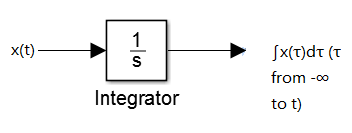····
因为积分器可以很方便的用放大器来实现, 就直接导致了模拟的实现, 这种实现既是早期模拟计算机
, 又是当今模拟计算机的基础.


奇异函数

    从另一个角度来审视冲激函数, 以便对这一重要的理想化信号得到进一步的认识.

1. 作为理想化段脉冲的单位冲激
x(t) = x(t) * δ(t);
因此, 如果取x(t) = δ(t), 就有
δ(t) = δ(t) * δ(t);

2. 通过卷积定义单位冲激
x(t) = x(t) * δ(t);
若令x(t) = 1;
1 = ∫δ(τ)dτ；
所以, 单位冲激的面积为1.

3. 单位冲激偶和其他奇异函数
单位冲激是一类称为奇异函数的信号中的一种, 其中每一种信号都是借助于它在
卷积运算中的作用来定义的.
考虑输入是输出的导数的线性时不变系统, 即:
y(t) = x'(t);
即有:
x'(t) = u1(t) * x(t);
u1(t), 单位冲激偶.
u1(t) * u(t) = δ(t);

考虑输入是输出的积分的线性时不变系统, 即:
y(t) = ∫x(τ)dτ (τ from -∞ to t)
即有:
∫x(τ)dτ = u(t) * x(t);
u-2(t) = u(t) * u(t) = ∫u(τ)dτ = tu(t), 单位斜坡函数;

u-1(t) * u1(t) = u0(t); (u-k(t): k个积分器级联, uk(t): k个微分器级联)

展开全文• 线性时不变系统特性 齐次性 若激励f(t)产生的响应为y(t)，则激励Af(t)产生的响应即为Ay(t)，此性质即为齐次性。其中A为任意常数。 f(t)系统y(t)，Af(t)系统Ay(t) 叠加性 若激励f1(t)与f2(t)产生的响应分别为y1(t)， ...
• 线性 线性linear，指量与量之间按比例、成直线的关系，在数学上...线性系统有两种定义：（1）根据系统的输入和输出关系是否具有线性来定义 满足叠加原理的系统具有线性特性。即若对两个激励x1(n)和x2(n)，有T[ax1
• 一个线性时不变（LTI）离散时间系统有以下四种描述方式 单位脉冲响应：系统在输入为单位脉冲信号时的输出响应 如一个滑动平均滤波器h(n)=[0.2，0.2，0.2]h(n) = [0.2，0.2， 0.2]h(n)=[0.2，0.2，0.2] 差分方程...脉冲响应 z变换 频率响应 差分方程
• 线性时不变系统对复指数信号的响应 特征函数以及特征值定义： 证明复指数信号是LTI系统的特征函数 简单运用上述性质 序言 复指数信号有相比于其他信号优良的性质，这使得其在数字信号处理以及信号与系统中...
• 注：本博客是基于奥本海姆《离散时间信号处理》第三版编写，主要是为了自己学习的复习与加深。 一、LTI系统的频率响应 ...其中||代表系统的幅度响应或增益，而为系统的相位响应或相移。式5.1和式5.2所表...
• 这是原先写在博客园的，原标题为“Linux Ubuntu 虚拟机系统自定义桌面分辨率且重启后保持不变”，现在做部分修改，适用于 Debian 系发行版： 我用 VMware Workstation 12 Pro 安装的 Ubuntu MATE Desktop ...Ubuntu Debian 分辨率 虚拟机 VMWare
• ，求其经过线性时不变系统的输出，假设线性时不变系统的系统函数为 这就证明了连续复指数信号 是LTI系统的特征函数，而常数 就是与特征函数 有关的特征值。 下面证明离散复指数信号 是LTI系统的特征函数： ...
• (1)如果系统从点x出发，那么系统未来的状态位置一直保持不变，一直待在出发点x，那么出发点x就叫做系统不变点。 (2)如果系统从某个区域内或者曲线出发上，例如以0为原点的半径为r的圆的区域或者有限环，系统的...
• 设：系统运动轨迹为，其中是初始点。 假设存在一个集合，其中 是一个实函数。 如果集合满足：初始点，且对于有。则称集合是一个正不变集。 形象的理解如下图所示： 圆锥是个正不变集，圆球是一个有初速度的...
• ## 旋转不变性

千次阅读 2014-09-17 14:01:09
在数学里，给予一个定义于内积空间的函数，假若对于任意旋转，函数的参数值可能会改变，但是函数的数值仍旧保持不变，则称此性质为旋转不变性（rotational invariance），或旋转对称性（rotational symmetry），...
• 最近想复习一下现代控制理论，在B站看到了DR_CAN大神做的视频，但对其中涉及的拉塞尔不变性定理理解地不太好，特地查了一下，写下来与君分享。 1、介绍 拉塞尔不变性定理是对Lyapunov直接法(Lyapunov第二法)的补充...控制理论
• ## 什么是真正的实时操作系统

万次阅读 多人点赞 2018-07-19 14:40:34
做嵌入式系统开发有一段时间了，做过用于手机平台的嵌入式Linux，也接触过用于交换机、媒体网关平台的VxWorks，实际应用后回过头来看理论，才发现自己理解的肤浅，也发现CSDN上好多同学们都对实时、嵌入式这些概念...
• ## 标准Web系统的架构分层

万次阅读 多人点赞 2015-06-22 10:30:36
标准Web系统架构适用于传统的基于WEB浏览器/手机端的CRM系统、ERP系统、SaaS系统、O2O系统、商城系统、物流系统。架构的灵活性和业务适应性决定了不同的系统根据业务形态、访问量、安全性所作出的架构设计也是不一样...架构设计
• 个人博客Glooow，欢迎各位老师来踩踩 文章目录1. 系统模型2. 基本定义3. 拉萨尔不变性原理 ...其中 f(0)=0f(0)=0f(0)=0。 2. 基本定义 正极限点(positive limit point)：p 被称为 x(t)x(t)x(t) 的正极限点，...
• 基于梯度调整的矩不变自动阈值图像分割算法来源：电子技术应用 作者：罗诗途 罗飞路 张王已 王艳玲摘要：提出了一种改进的矩不变自动阈值算法。该算法针对矩不变自动阈值法忽略图像细节的缺点，在矩不变自动阈值的...算法 工具 网络
• Java多线程编程中，常用的多线程设计模式包括：Future模式、Master-Worker模式、Guarded Suspeionsion模式、不变模式和生产者-消费者模式等。这篇文章主要讲述……，关于其他多线程设计模式的地址如下： 关于Future...Java 多线程 设计模式
• 一、线性反馈系统1、连续时间线性时不变反馈系统的一般结构可以用图11.3(a)所示，而离散时间线性时不变反馈系统则可以用图11.3(b)来表示由于反馈系统最典型的应用场合都是在因果系统中，所以和那自然把图11.3(a)和图...
• 颜色不变性定义：室外光线的彩色成分变化非常大，但人却能正确的感知场景中物体的颜色，并且在大部分情况下不依赖于环境照明的颜色，这种现象叫彩色不变性   成像设备在获取并记录图像时，只能获得场景或物体在...
• 对于每一个Linux学习者来说，了解Linux文件系统的目录结构，是学好Linux的至关重要的一步.，深入了解linux文件目录结构的标准和每个目录的详细功能，对于我们用好linux系统只管重要，下面我们就开始了解一下linux...linux
• ），将其中的小写英文 字母变换为大写英文字母，其他字符保持不变。然后将变换后的字符串显示出来。 data segment buf db 101,?,101 dup(?) data endsstack segment stack dw 20h dup(?) top label word stack ends...汇编语言
• ## 支付系统-对账系统

万次阅读 多人点赞 2019-04-08 14:17:04
在支付系统中，资金对账在对账中心进行，将系统保存的账务流水与银行返回的清算流水和清算文件进行对账，核对系统账务数据与银行清算数据的一致性，保证支付机构各备付金银行账户每日的预计发生额与实际发生额一致。...对账中心
• 不变模式(Immutable Pattern)顾名思义,它的状态在它的生命周期内是永恒的(晕,永恒的日月星晨,对象如人, 太渺小,谈不上永恒!... 请注意上面这段话,不变模式其中不变类,说明不变类只是不变模式中一java string immutable jvm object
• 本节主要内容如下自由响应与强迫响应自由响应是反应的系统的固有频率，和系统的输入没有关系。是齐次解。当然，虽然齐次解和输入信号无关，但是齐次解前面的系数和输入信号有关。强迫响应是系统的特解，与输入信号......